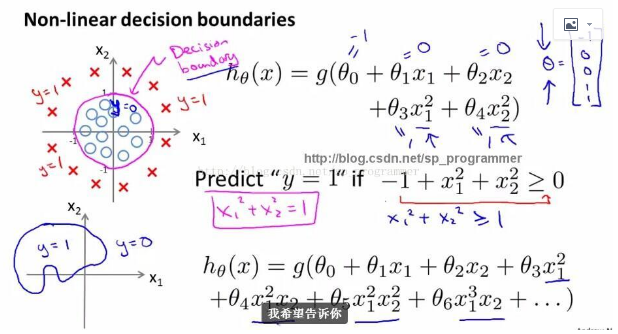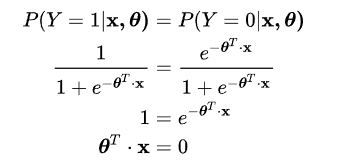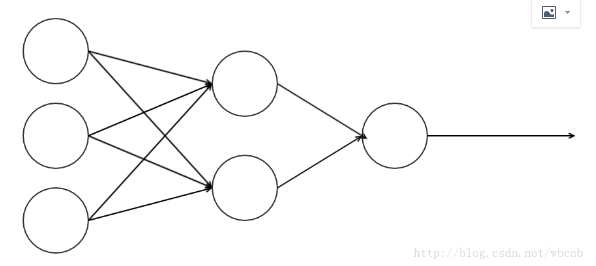# 线性模型和非线性模型的区别？

a. 线性模型可以用曲线拟合样本，线性模型可以是用曲线拟合样本，但是分类的决策边界一定是直线的，例如logistics模型（广义线性模型，将线性函数进行了一次映射）。

b. 区分是否为线性模型，主要是看一个乘法式子中自变量x前的系数w,如果w只影响一个x，那么此模型为线性模型。或者判断决策边界是否是线性的

$y=\frac{1}{1+e^{w_0+w_1*x_1+w_2*x_2}}$

$y=\frac{1}{1+w_5*e^{w_0+w_1*x_1+w_2*x_2}}$

c. 其实最简单判别一个模型是否为线性的，只需要判别决策边界是否是直线，也就是是否能用一条直线来划分

## 引申问题: 神经网络激励函数的作用是什么？# 举例：

## 线性模型：LR

Logistic Regression模型是广义线性模型，因为 logistic 回归的决策边界（decision boundary）是线性的：LR是线性模型，可以理解为将普通的线性模型的结果映射到一个（0,1）的区间而已，然后划分一个界限（0.5）

## 非线性模型：神经网络$y1=\frac{1}{1+e^{w_0+w_1*x_1+w_2*x_2+w_3*x_3}}$
$y2=\frac{1}{1+e^{w_4+w_5*x_1+w_6*x_2+w_7*x_3}}$

$z=\frac{1}{1+e^{k_1+k_2*y_1+k_3*y_2}}$

posted @ 2018-03-15 16:24 ToOnE 阅读( ...) 评论( ...) 编辑 收藏
09-07
04-18549708-085258
02-26
12-151万+
11-021万+
10-211万+
09-263139
04-162018
02-22710